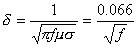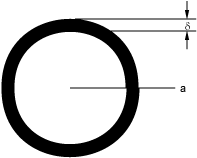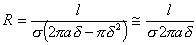# Skin Depth/Skin Effect and Calculator

Because high frequency signals do not penetrate well into good conductors, the resistance associated with a conductor at these high frequencies will be higher than the dc resistance. This effect is know as the skin effect since the high frequency current flows in a thin layer near the surface of the conductor. The formula to determine the effective skin depth for a conductor is shown below.Equation to calculate the skin depth or skin effect of a copper conductor

for pure copper
In this case, d is the skin depth (in m), f is the frequency of interest (in Hz), m is the permeability of the material (mo, or 1.2566E-6 H/m for most materials),s is the conductivity of the material (in Siemens/m or 1/r where r is the resistivity in ohm-m).Diagram to show effective resistance of a circular wire due to skin effect

If a circular wire is used with radius a, the effective resistance of the wire can be calculated as shown below where l is the length of the wire and the other variables are defined as above.Equation to calculate the effective resistance of a wire due to skin depth

when a >> d

The above equation applies for those cases where the skin depth is between 0 and the wire radius, a. If the skin depth is larger than the wire radius, then the equivalent ac resistance of the wire is no different than the dc resistance and is merely determined by the standard formula using the entire wire cross-sectional area. As the frequency approaches zero (dc), the skin depth becomes infinite and as the freqency increases, the skin depth becomes smaller and smaller.

The following table shows how the skin depth varies with some example conductor materials (pure copper and pure aluminum) for typical pulsed power and power conditioning frequencies in the range of 1 kHz to 1 GHz.

 Frequency (Hz) Skin Depth in Copper (cm) Skin Depth in Aluminum (cm) 1000 2.09E-1 2.68E-1 10,000 6.61E-2 8.46E-2 100,000 2.09E-2 2.68E-2 1,000,000 6.61E-3 8.46E-3 10,000,000 2.09E-3 2.68E-3 100,000,000 6.61E-4 8.46E-4 1,000,000,000 2.09E-4 2.68E-4

The calculator below can be used to determine the skin depth for a given material alloy and operating frequency. It will also display the resistivity, conductivity, and permeability assumed for the chosen material. It is suggested that users double check this information since material resistivity can vary depending upon the exact composition, tempering, etc. of the selected material alloy. Credit for the initial Javascript code used in the calculator is given to Ray Allen who has a number of similar useful calculators on his website, Pulsed Power Portal.

Calculate Skin Depth

 Parameter Value Units Inputs: f (Frequency) kHzMHzGHzHz____________________ Material Alloy Pure AluminumAluminum 3105Aluminum 5005Aluminum 5052Aluminum 6061Aluminum 6063Aluminum 6101Pure CopperCopper C10100Copper C10200Copper C10300Copper C10400Copper C10700Copper C11000Copper C11300Copper C11400Admiralty BrassCartridge BrassNaval BrassRed BrassYellow BrassCommercial BronzeBerylliumChromiumGraphiteGoldNickelSilverTungsten____________________ m (Rel Permeability) Outputs: s (Conductivity) Siemens/mSiemens/cmSiemens/mm____________________ r (Resistivity) ohm-mkohm-mMohm-mGohm-mmohm-muohm-mohm-cmkohm-cmMohm-cmGohm-cmmohm-cmuohm-cm____________________ d (Skin Depth) mcmmminmil____________________

Output Format:

 Select Format: Scientific Engineering Fixed

Send consulting inquiries, comments, and suggestions to richard.ness@nessengr.com